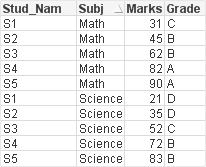QlikView App Development

Discussion Board for collaboration related to QlikView App Development.

Contributor

Extended IntervalMatch

Hello all,

Below is my script for extended interval match and the expected output. its simple not working and I can't figure out why. Could you please help?

Range:
1, 20, D,Math
21, 40, C,Math
41, 80, B,Math
81, 100, A,Math
1, 40, D,Science
41, 60, C,Science
61, 90, B,Science
91, 100, A,Science
]
;

Marks:
Stud_Nam,Marks, Subj
S1,31,Math
S2,45,Math
S3,62,Math
S4,82,Math
S5,90,Math
S1,21,Science
S2,35,Science
S3,52,Science
S4,72,Science
S5,83,Science
]
;
Inner join
IntervalMatch(Marks,Subj) load [Max range] as MaxRange, [Min Range] as MinRange resident Range;

expected output:

 Stud_Nam Subj Marks Grade S1 Math 31 C S2 Math 45 B S3 Math 62 B S4 Math 82 A S5 Math 90 A S1 Science 21 D S2 Science 35 D S3 Science 52 C S4 Science 72 B S5 Science 83 B

Tags (1)
1 Solution

Accepted SolutionsMVP

Re: Extended IntervalMatch

Range:

1, 20, D,Math

21, 40, C,Math

41, 80, B,Math

81, 100, A,Math

1, 40, D,Science

41, 60, C,Science

61, 90, B,Science

91, 100, A,Science

];

Marks:

Stud_Nam,Marks, Subj

S1,31,Math

S2,45,Math

S3,62,Math

S4,82,Math

S5,90,Math

S1,21,Science

S2,35,Science

S3,52,Science

S4,72,Science

S5,83,Science

];

Inner join

IntervalMatch(Marks,Subj) load [Max range], [Min Range], Subj resident Range;

LEFT JOIN

DROP TABLE Range;

6 Replies
Honored Contributor III

Re: Extended IntervalMatch

It should be:

Inner join
IntervalMatch(Marks,Subj) load [Max range] as MaxRange, [Min Range] as MinRange, Subj resident Range;

drop Table Range;

May you live in interesting times!MVP

Re: Extended IntervalMatch

Range:

1, 20, D,Math

21, 40, C,Math

41, 80, B,Math

81, 100, A,Math

1, 40, D,Science

41, 60, C,Science

61, 90, B,Science

91, 100, A,Science

];

Marks:

Stud_Nam,Marks, Subj

S1,31,Math

S2,45,Math

S3,62,Math

S4,82,Math

S5,90,Math

S1,21,Science

S2,35,Science

S3,52,Science

S4,72,Science

S5,83,Science

];

Inner join

IntervalMatch(Marks,Subj) load [Max range], [Min Range], Subj resident Range;

LEFT JOIN

DROP TABLE Range;

Highlighted
Honored Contributor III

Re: Extended IntervalMatch

May be this

Range:
LOAD *,[Max range]+IterNo()-1 as Marks INLINE [
1, 20, D,Math
21, 40, C,Math
41, 80, B,Math
81, 100, A,Math
1, 40, D,Science
41, 60, C,Science
61, 90, B,Science
91, 100, A,Science
]
While [Max range]+IterNo() <= [Min Range];

Marks:
Inner Join
Stud_Nam,Marks, Subj
S1,31,Math
S2,45,Math
S3,62,Math
S4,82,Math
S5,90,Math
S1,21,Science
S2,35,Science
S3,52,Science
S4,72,Science
S5,83,Science
]
;Contributor

Re: Extended IntervalMatch

Thanks Antonio. This exactly fetches me the desired result.

Is this scenario shouldn't be done using interval match at all?

Honored Contributor III

Re: Extended IntervalMatch

I prefer this way.

Intervalmatch creates table Bridge between Your Tables.

IterNo() generates Rows in Table.

However Stefan's answer is correct way to use Intervalmatch.

Honored Contributor III

Re: Extended IntervalMatch

Downside of this method is that you can get a huge table in memory before your join where the table you created for the interval match stays tiny.

Your current example is only with a limited number of values, but imagine the change in size of the table between the two methods when you get a lot more values to match.

So to save memory during the reload and likely higher performance, I would still stick to the interval match.

If the amount of matches stays tiny and performance is far from an issues, it doesn't matter of course.

May you live in interesting times!